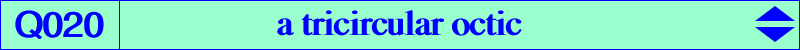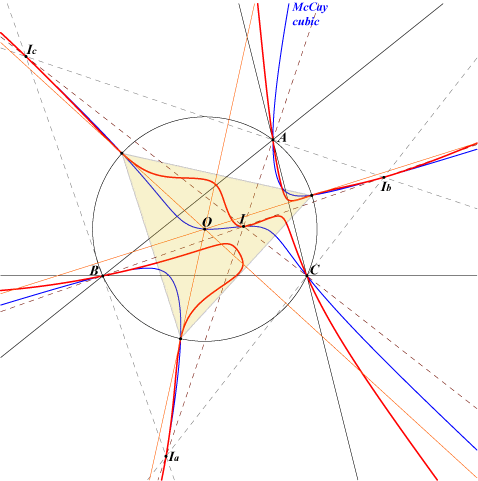too complicated to be written here. Click on the link to download a text file.X(1), X(2574), X(2575) excenters X(2574), X(2575) : points at infinity of the Jerabek hyperbola vertices of the circumnormal triangle (on the McCay cubic) foci of the inconic with center O, perspector X(69) isogonal conjugates of the Ix-anticevian points, see Table 23Let P be a point and let N', N" be the nine-point centers of the pedal and antipedal triangles of P respectively. P, N', N" are collinear if and only if P lies on the octic Q020 or on the line at infinity. Q020 is a tricircular octic with two real asymptotes parallel to those of the Jerabek hyperbola. The tangents at the in/excenters and at the vertices of the circumnormal triangle pass through O. The points A, B, C and the circular points at infinity are triple points on the curve.